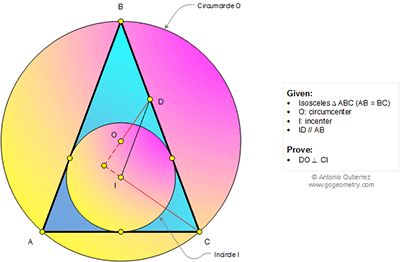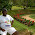## Tuesday, July 7, 2015

### Geometry Problem 1129: Isosceles Triangle, Circumcenter, Incenter, Parallel Lines, Perpendicular Lines

Geometry Problem. Post your solution in the comments box below.
Level: Mathematics Education, High School, Honors Geometry, College.

Click the diagram below to enlarge it.#### 3 comments:

1.Let DO & CI, both extended, meet in X. /_AOC=2B since O is the circumcentre and thus /_COI=/_B. Since DI//BA, /_CDI=/_B. Quad CDOI is, therefore, concyclic and thus, /_IOX=/_DCI=/_C/2.
We note that /_OIX=90° - /_C/2 which, in turn, means that /_DXI =180° - (90-C/2) - C/2 = 90°. QED.

1."Quad CDOI is, therefore, concyclic"
How does it follow from your previous statements?

2.< IOC = < B from Tr. BOC = < IDC since ID //AB. So IODC is cyclic and so < MOI = < ICD = < A/2 = < ICN.

Hence MOCN is cyclic and so < OMC = < ONC = 90.

Note - M is where DO meets CI and N is the mid point of AC (note that B, O, I, N are all collinear since Tr. ABC is isoceles)

Sumith Peiris
Moratuwa
Sri Lanka